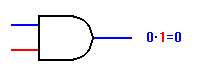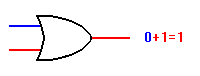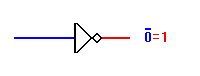# Basic Logical Functions and GatesWhile each logical element or condition must always have a logic value of either "0" or "1", we also need to have ways to combine different logical signals or conditions to provide a logical result.

For example, consider the logical statement: "If I move the switch on the wall up, the light will turn on." At first glance, this seems to be a correct statement. However, if we look at a few other factors, we realize that there's more to it than this. In this example, a more complete statement would be: "If I move the switch on the wall up and the light bulb is good and the power is on, the light will turn on."

If we look at these two statements as logical expressions and use logical terminology, we can reduce the first statement to:

Light = Switch

This means nothing more than that the light will follow the action of the switch, so that when the switch is up/on/true/1 the light will also be on/true/1. Conversely, if the switch is down/off/false/0 the light will also be off/false/0.Looking at the second version of the statement, we have a slightly more complex expression:

Light = Switch and Bulb and Power

Normally, we use symbols rather than words to designate the and function that we're using to combine the separate variables of Switch, Bulb, and Power in this expression. The symbol normally used is a dot, which is the same symbol used for multiplication in some mathematical expressions. Using this symbol, our three-variable expression becomes:

 Light = Switch . Bulb . PowerWhen we deal with logical circuits (as in computers), we not only need to deal with logical functions; we also need some special symbols to denote these functions in a logical diagram. There are three fundamental logical operations, from which all other functions, no matter how complex, can be derived. These functions are named and, or, and not. Each of these has a specific symbol and a clearly-defined behavior, as follows:The AND Gate The AND gate implements the AND function. With the gate shown to the left, both inputs must have logic 1 signals applied to them in order for the output to be a logic 1. With either input at logic 0, the output will be held to logic 0. If your browser supports the Javascript functions required for the demonstrations built into this page, you will see buttons to the left of the AND gate drawing. You can click on these to change their assigned logic values, and the drawing will change to reflect the new input states. There is no limit to the number of inputs that may be applied to an AND function, so there is no functional limit to the number of inputs an AND gate may have. However, for practical reasons, commercial AND gates are most commonly manufactured with 2, 3, or 4 inputs. A standard Integrated Circuit (IC) package contains 14 or 16 pins, for practical size and handling. A standard 14-pin package can contain four 2-input gates, three 3-input gates, or two 4-input gates, and still have room for two pins for power supply connections.The OR Gate The OR gate is sort of the reverse of the AND gate. The OR function, like its verbal counterpart, allows the output to be true (logic 1) if any one or more of its inputs are true. Verbally, we might say, "If it is raining OR if I turn on the sprinkler, the lawn will be wet." Note that the lawn will still be wet if the sprinkler is on and it is also raining. This is correctly reflected by the basic OR function. In symbols, the OR function is designated with a plus sign (+). In logical diagrams, the symbol to the left designates the OR gate. As with the AND function, the OR function can have any number of inputs. However, practical commercial OR gates are mostly limited to 2, 3, and 4 inputs, as with AND gates.The NOT Gate, or Inverter The inverter is a little different from AND and OR gates in that it always has exactly one input as well as one output. Whatever logical state is applied to the input, the opposite state will appear at the output. The NOT function, as it is called, is necesasary in many applications and highly useful in others. A practical verbal application might be: The door is NOT locked = You may enter The NOT function is denoted by a horizontal bar over the value to be inverted, as shown in the figure to the left. In some cases a single quote mark (') may also be used for this purpose: 0' = 1 and 1' = 0. For greater clarity in some logical expressions, we will use the overbar most of the time. In the inverter symbol, the triangle actually denotes only an amplifier, which in digital terms means that it "cleans up" the signal but does not change its logical sense. It is the circle at the output which denotes the logical inversion. The circle could have been placed at the input instead, and the logical meaning would still be the same.